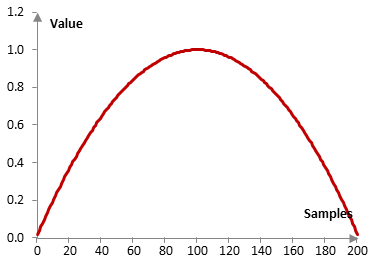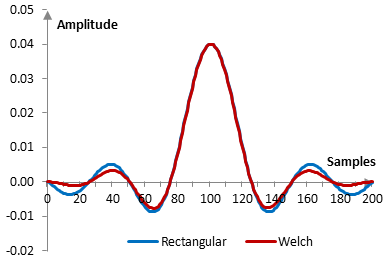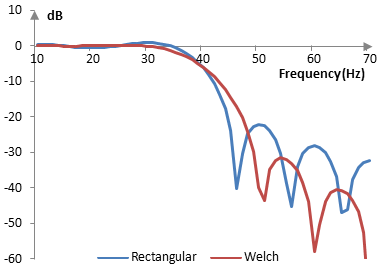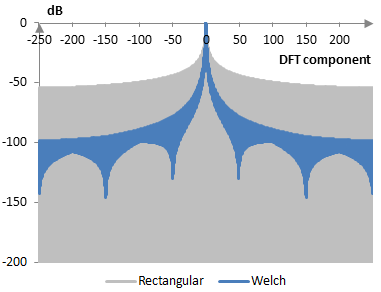# Welch window

The Welch window coefficients are given by the following formula

$$a(k)=1-(\frac{k-\frac{N-1}{2}}{\frac{N+1}{2}})^2$$

where N is the length of the filter and k = 0, 1, …, N – 1.

The Welch window is a quadratic polynomial and a parabola.

Consider a finite impulse response (FIR) low pass filter of length N = 201. The following is the Welch window.Given a sampling frequency of 2000 Hz and a filter cutoff frequency of 40 Hz, the impulse response of the filter with a rectangular window (with no window) and with the Welch window is as follows.The magnitude response of the same filter is shown on the graph below.## Measures for the Welch window

The following graph compares the discrete Fourier transform of the Welch window with that of the rectangular window.The Welch window measures are as follows.

 Coherent gain 0.67 Equivalent noise bandwidth 1.20 Processing gain -0.78 dB Scalloping loss -2.23 dB Worst case processing loss -3.02 dB Highest sidelobe level -21.3 dB Sidelobe falloff -11.0 dB / octave, -36.5 dB / decade Main lobe is -3 dB 1.16 bins Main lobe is -6 dB 1.58 bins Overlap correlation at 50% overlap 0.345 Amplitude flatness at 50% overlap 0.670 Overlap correlation at 75% overlap 0.765 Amplitude flatness at 75% overlap 0.910

Window

### Filtered HTML

• Freelinking helps you easily create HTML links. Links take the form of [[indicator:target|Title]]. By default (no indicator): Click to view a local node.